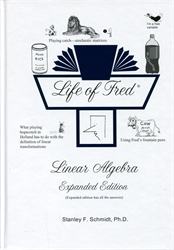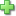# Life of Fred: Linear Algebra

Price: \$52.00

There are many different "algebras":

• Beginning Algebra = Algebra 1 = first year of high school algebra
• Advance Algebra = Algebra 2 = second year of high school algebra
• Linear Algebra (which is what this book is about)
• Modern Algebra = Abstract Algebra which is usually studied as an upper-division course at the university. It deals with groups, rings, fields, . . . (whatever those are!)
• Boolean Algebra
• and many more algebras

Life of Fred: Linear Algebra is usually studied after the two years of college calculus. You will study:Systems of equations with lots of ways to solve themAll kinds of spaces: Vector, Inner Product, and Dual SpacesLinear Transformations including linear functionals.

Here's a more complete description:

Chapter 1 Systems of Equations with One Solution

high school algebra, three equations with three unknowns
coefficient and augmented matrices
elementary row operations
Gauss-Jordan elimination
Gaussian elimination

Chapter 1½ Matrices

scalar multiplication rA
matrix multiplication AB
matrix inverse A–1
proof of associative law of matrix multiplication (AB)C = A(BC)
elementary matrices
LU-decomposition
permutation matrices

Chapter 2 Systems of Equations with Many Solutions

four difficulties with Gauss-Jordan elimination
#1: a zero on the diagonal
#2: zeros “looking south”
#3: zeros “looking east”
#4: a row with all zeros except for the last column
free variables
echelon and reduced row-echelon matrices
general solutions
homogeneous systems
rank of a matrix

Chapter 2½ Vector Spaces

a very short course in abstract algebra
four properties of scalar multiplication
five vector spaces
linear combinations and spanning sets
linear dependence/independence
basis for a vector space
coordinates with respect to a basis
dimension of a vector space
subspace of a vector space
row space, column space, null space, and nullity

Chapter 2¾ Inner Product Spaces

dot product
inner product
positive-definiteness
length of a vector (norm of a vector)
angle between two vectors
perpendicular vectors (orthogonality)
Gram-Schmidt orthogonalization process
orthonormal sets
Fourier series
harmonic analysis
double Fourier series
complex vector spaces with an inner product
orthogonal complements

Chapter 3 Systems of Equations with No Solution

overdetermined/underdetermined systems
discrete/continuous variables
the normal equation/“the best possible answer”
least squares solution
data fitting
model functions

Chapter 3½ Linear Transformations

rotation, reflection, dilation, projection, derivatives, matrix multiplication
linear transformations, linear mappings, vector space homomorphisms
linear operators
ordered bases
zero transformation, identity transformation
the equivalence of linear transformations and matrix multiplication
Hom(V , W )
linear functionals
dual spaces
second dual of V

Chapter 4 Systems of Equations into the Future

transition matrix
determinants
characteristic polynomial/characteristic equation
eigenvalues
algebraic multiplicity/geometric multiplicity
computation of A100
stochastic matrices
Markov chains
regular matrices
absorbing states
similar matrices
systems of linear differential equations
Fibonacci numbers
computer programs for linear algebra

Index

Six problems sets at the end of each chapter.Click here to write a review